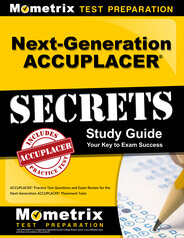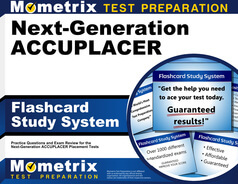Next Generation ACCUPLACER Advanced Algebra and Functions Practice Test

The Next Generation ACCUPLACER advanced algebra and functions subtest is a computer-adaptive test intended to evaluate individual test takers’ capabilities in order to place them into appropriate classes for their skill and knowledge level. This test is appropriate for students whom are either undecided in their major or are entering into many non-STEM fields of study. The 20-question, multiple-choice test is computer adaptive, meaning the test will automatically select a suitable difficulty level as you progress through the test based on your previous responses.

 Next-Gen ACCUPLACER Test Next-Gen ACCUPLACER Study Guide Next-Gen ACCUPLACER Flashcards

Calculator Use

You will not be permitted to bring a calculator into the testing location or use one on the ACCUPLACER math test. If you are presented any questions which have been configured to permit the use of a calculator, you will be presented with an icon on the screen which will grant you access to an on-screen calculator for that question.

How to Make an Appointment

The ACCUPLACER math test is administered by College Board. When you are ready to schedule an appointment to take the test, you may visit your institution’s advising or counseling center for instruction. They will be able to guide you through the necessary steps and process to register for an appointment both in-state or out-of-state, depending on your institution. If your institution is out-of-state, you will need to take additional steps to receive permission and schedule your appointment with remote testing options.

Next Generation ACCUPLACER Advanced Algebra and Functions (AAF) Subtest

The 20-question Next Generation ACCUPLACER advanced algebra and functions (AAF) subtest will assess your capabilities with the following knowledge and skills:

• Exponential and Logarithmic Equations (1-3 questions / 5-15%)
• Creating Exponential and Logarithmic Equations in One and Two Variables
• Graphing Exponential and Logarithmic Equations
• Interpreting Exponential and Logarithmic Equations
• Solving Exponential and Logarithmic Equations
• Factoring (1-2 questions / 5-10%)
• Factoring Methods Applied to Quadratics, Cubics and Polynomials
• Functions (2-4 questions / 10-20%)
• Creating Functions Using Function Notation
• Evaluating Linear and Quadratic Functions
• Graphing Functions
• Interpreting Functions within a Context
• Geometry Concepts for Algebra I (1-2 questions / 5-10%)
• Creating Expressions for Area, Perimeter and Volumes
• Evaluating Dilations, Rotations, Translations and Reflections
• Using Distance Formula and Pythagorean Theorem
• Geometry Concepts for Algebra II (1-2 questions / 5-10%)
• Determining Volume of Nonprism Objects
• Using Circle Equations in the Coordinate Plane
• Using Intersecting Line Theorems
• Using Triangle Similarity and Congruency Theorems
• Linear Applications and Graphs (2-3 questions / 10-15%)
• Applying Linear Equations to Real-Life Contexts
• Graphing Linear Equations in Two Variables
• Linear Inequalities
• Parallel and Perpendicular Lines
• Systems of Equations
• Using Elementary Linear Functions for Describe Relationships
• Linear Equations (2-3 questions / 10-15%)
• Creating Linear Equations in One or Two Variables
• Simplifying Linear Equations and Inequalities
• Solving Linear Equations
• Solving Systems of Two Linear Equations
• Polynomial Equations (1-3 questions / 5-15%)
• Creating Polynomial Equations in One and Two Variables
• Graphing Polynomial Equations
• Solving Polynomial Equations
• Quadratics (2-3 questions / 10-15%)
• Creating Quadratic Equations in One or Two Variables
• Simplifying Quadratic Equations and Inequalities
• Solving Quadratic Equations
• Solving Systems that Involve a Quadratic Equation
• Radical and Rational Equations (1-3 questions / 5-15%)
• Creating Radical and Rational Equations and Functions in One Variable
• Determining Domain and Range for Radical and Rational Functions
• Graphing Radical and Rational Functions
• Simplifying Radical and Rational Expressions and Equations
• Trigonometry (1-3 questions / 5-15%)
• Determining Arc Length and Radian Measures
• Evaluating Equivalent Trigonometric Functions
• Graphing Trigonometric Relationships
• Solving Trigonometric Equations
• Using the Law of Sines and the Law of Cosines
• Using Right Triangle Trigonometry (including special triangles)

Preparing for the ACCUPLACER Math Test

As the ACCUPLACER math test is intended to help place you into appropriate classes based on your individual level, you should prepare for the test by using resources available to you in order to be able to perform at your best. Mometrix has a free ACCUPLACER test prep library, which includes comprehensive study resources such as ACCUPLACER practice tests and ACCUPLACER study guides to help you review these critical concepts before test day.

Next Generation ACCUPLACER Study Guide

Next Generation ACCUPLACER FlashcardsACCUPLACER Next Generation Test

by Mometrix Test Preparation | Last Updated: May 13, 2019